## Eigenvalue and Eigenvector of Symmetric Matrix

Symmetric matrix is a special type of square matrix that often come in many applications such as covariance matrix, correlation matrix and distance matrix. In this page, you will learn more about the eigenvalues and eigenvectors of symmetric matrix.

Symmetric matrix is matrix where the transpose is equal to itself, that is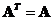. Symmetric matrix has a special property that the eigenvalues of symmetric matrix is always real number (remember that eigenvalues can be complex number). This greatly simplifies many applications because you do not need to handle complex number arithmetic.

Furthermore, symmetric matrix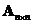also has another special property that the eigenvectors are linearly independent . If we can find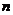linearly independent eigenvectors, these eigenvectors are the right candidate for basis vectors to create new space indicated by new coordinate system. Not only that, there is another property of symmetric matrix that if the eigenvalues of symmetric matrix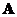are distinct (all eigenvalues are different with no multiple eigenvalues), then the eigenvectors that belong to distinct eigenvalues are orthogonal . This is a very nice property because using symmetric matrix you will get basis vectors, and these basis vectors are guaranteed to be perpendicular to each other. Some applications such as Principal Component Analysis (PCA) and Multi Dimensional Scaling (MDS) rely heavily on these properties.

Lastly but not least, if you can find that all eigenvalues of a symmetric matrixare distinct (all the eigenvalues are simple), then matrixcan be transformed into a diagonal matrix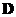simply by formula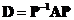. Modal matrix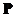is formed by horizontal concatenation of thelinearly independent eigenvectors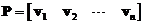. Since the matrixis symmetric, its modal matrix is orthogonal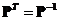. The eigenvalues oflie on the main diagonal of. Diagonal matrix simplified much computation because it behaves almost similar to scalar. For example, you can take any function such as power or exponent and even derivative only to the diagonal elements.

The interactive program below helps you to find eigenvalues and eigenvectors of a symmetric matrix. The program is using Jacobi method to compute symmetric Eigen system. The rational output is an approximation of the decimal format. When you click Random Example button, the program will generate random symmetric matrix. Practically, this Random Example gives you unlimited examples for you to investigate the behavior of eigenvalues and eigenvectors of a symmetric matrix. For instance, you may check that the eigenvectors are orthonormal (the norm of each row and each column is one). Can you find any example that the eigenvalues are multiple to each other (not all distinct)?

Report in rational format

Yes, this program is a free educational program!! Please don't forget to tell your friends and teacher about this awesome program!